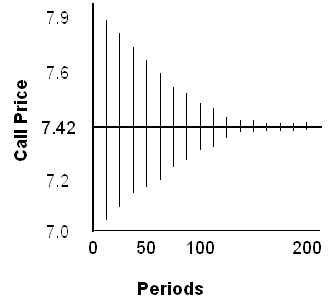### Why should I choose AnalystNotes?

Simply put: AnalystNotes offers the best value and the best product available to help you pass your exams.

### Subject 5. Extending the binomial model

We have seen how to generalize the binomial model to 2 and any number of periods. Increasing the number of periods allows for many possible stock price outcomes at expiration, thereby increasing the realism of the results.

To illustrate how the binomial model gives increasingly refined estimates as the number of periods increases, consider a European call option that has one year until expiration and an exercise price of \$100. Assume that the underlying stock trades for \$100, with a standard deviation of 0.1. The risk-free rate of interest is 6%. The graph below shows how the binomial prices converge to the true option price of \$7.42 as the number of periods increases. The binomial prices oscillate around the true price: for a single-period binomial model, the price is \$7.86. With two periods, the binomial model gives a price of \$7.18. With 20 periods the binomial price is 7.38, and with 100 periods the binomial price is 7.44.In general, for an option of a given expiration, a greater pricing accuracy is obtained by dividing the option's life into a greater number of time periods in a binomial tree. As more time periods are added, the discrete-time binomial price converges to a stable value as though the option is being modeled in a continuous-time world.